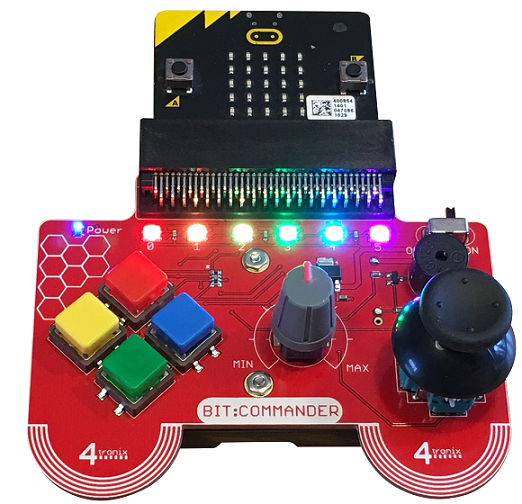# BBC micro:bit Bit:Commander Joystick

## Introduction

The joystick on the Bit:Commander is exactly the same type as you would find on a standard game controller, what you might call thumbsticks. These joysticks are effectively two potentiometers, one for the x axis and one for the y axis. Movement on each axis effects the reading on each of the potentiometers, allowing you to determine the direction and distance that the stick was moved. This means that you can use the joystick readings to make variable changes to parts of your program or circuit, like moving faster if the stick is pushed further forwards. Pressing the stick closes a pushbutton that can also be read.## Programming

This first program just prints the readings to the REPL window. Even if you don't have that, you can see how easy it is to read from the joystick.

```from microbit import *

while True:
print(x,y,j)
sleep(20)
```

### Dot Commander

Although the joystick is an analogue device, we can still use it like a switch if we think about how to code for it. The following program allows you to move a dot around the LED matrix, using the joystick.

```from microbit import *

# co-ordinate of the dot
x = 2
y = 2

# follower variables
# storing the previous change to x or y
# -1 0 1
lastdx = 0
lastdy = 0

while True:
# undraw the pixel in its current location
display.set_pixel(x,y,0)
# work out whether to change x or y and by how much
if dx<150:
dx = -1
elif dx>850:
dx = 1
else:
dx = 0
if dy<150:
dy = 1
elif dy>850:
dy = -1
else:
dy = 0
# only apply the changes if a
# change of input occurs
if dx!=lastdx:
x = x + dx
if dy!=lastdy:
y = y + dy
# easy way to keep x and y in range
x = max(0,min(x,4))
y = max(0,min(y,4))
# redraw the pixel
display.set_pixel(x,y,9)
# set the follower variables ready for the next iteration
lastdx = dx
lastdy = dy
sleep(10)
```

### Scaling

In the last program, we were using the joystick a little like a button. You may have noticed that the joystick gives a reading of 527/528, sometimes flicking between them, when the stick is at rest. That is slightly off-centre for a range of 0 to 1023, leaving a wider range of values on one side of each axis.

We can correct for this by writing some code to convert the readings. The following program takes the readings and rescales them so that they come in the range -100 to 100, with 0 being the centre. It also adds a small dead zone around the centre position so that small movements of the stick are discarded.

```# Joysticks have a low of 1 and a high of 1023.
# The centre is around 527/528.
# This program centres the joystick and adds a small dead zone.
# Readings are scaled from -100 to 100.
from microbit import *

# The 10 in the selection statements is the dead zone,
# +-10 around the centre. Reads 0 when not moved.
# The 100 in the return statements is the scaling factor.